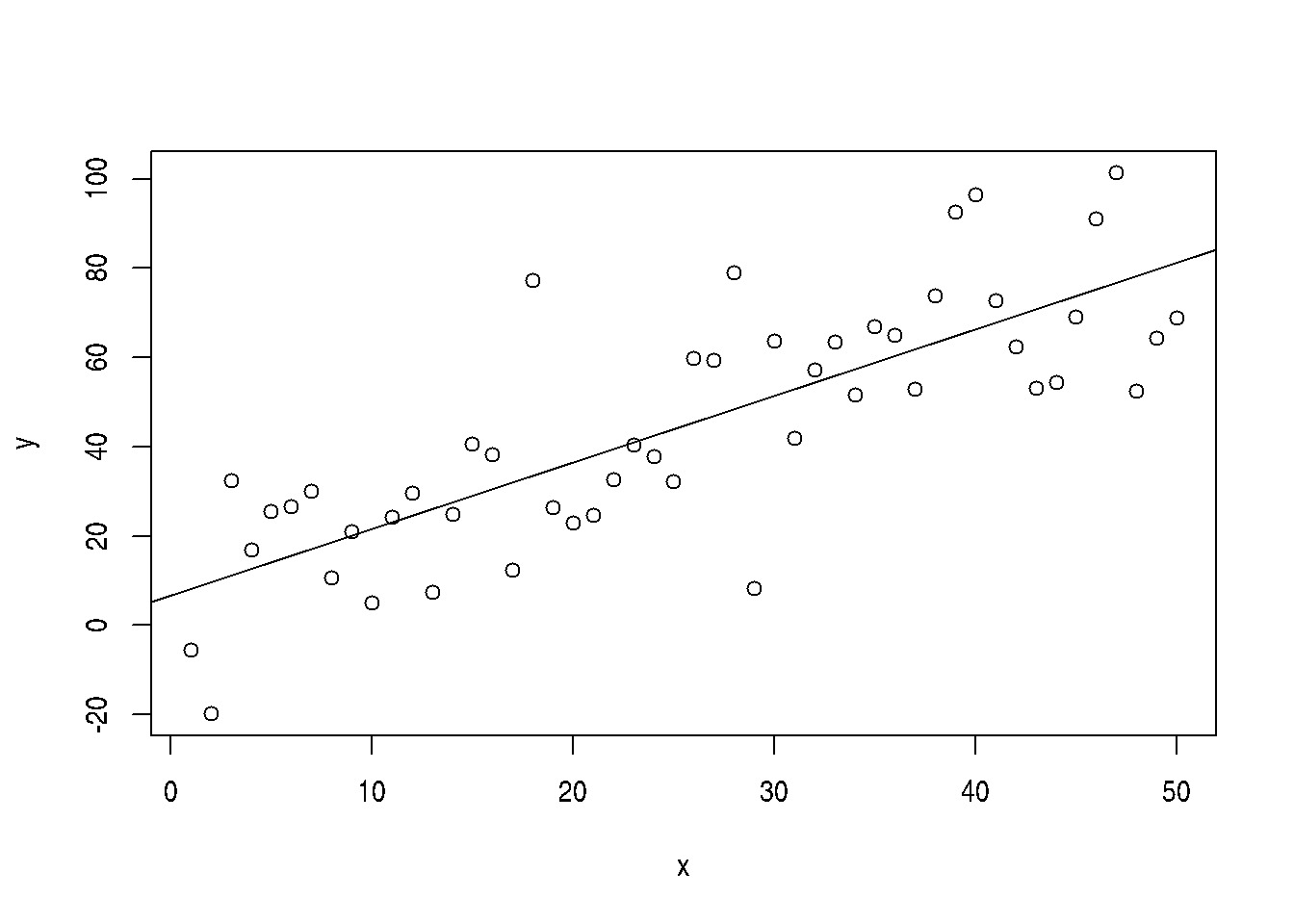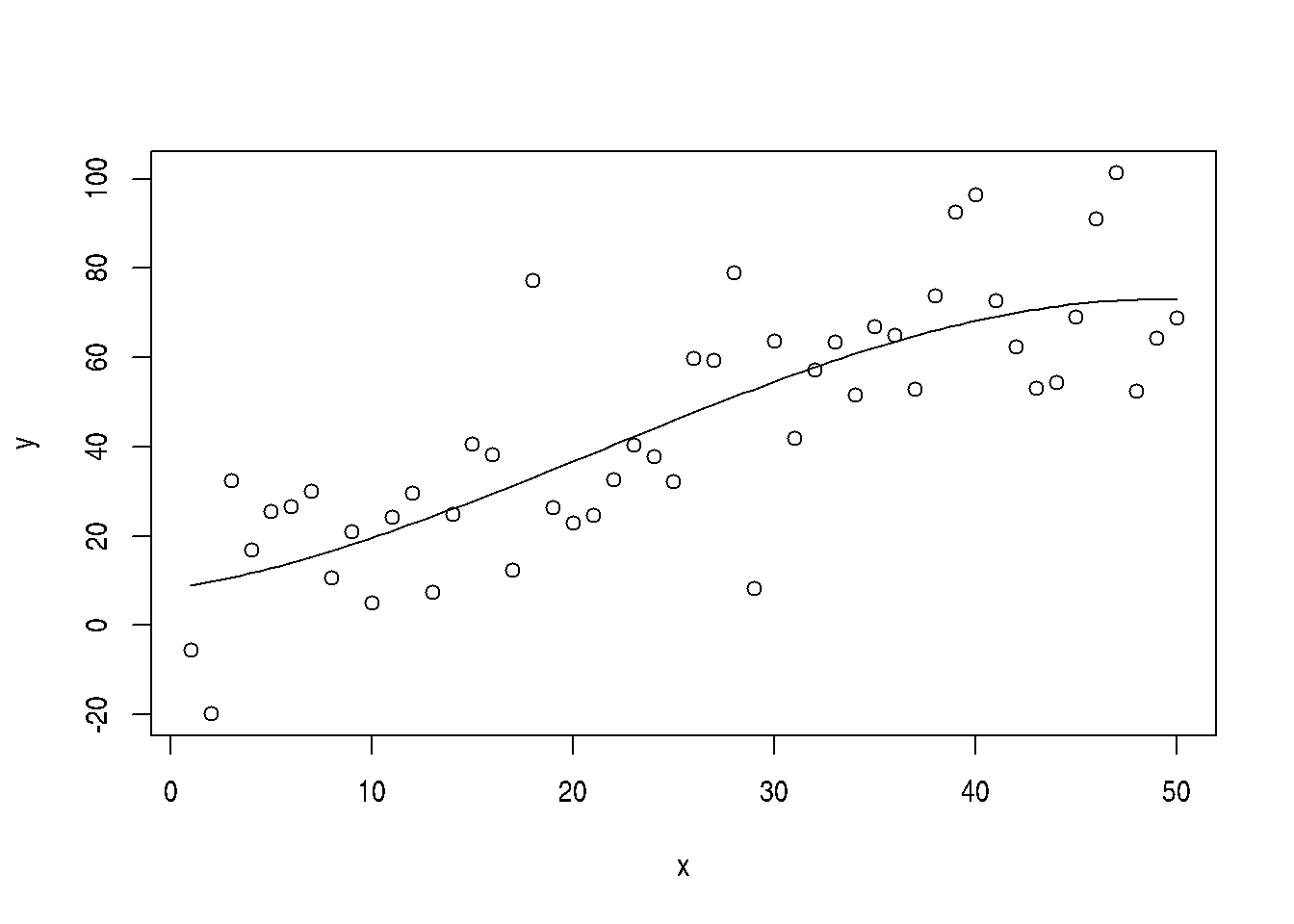# 2 R

The version of R used to make this document is 4.2.1. The version of the rmarkdown package used to make this document is 2.16. The version of the knitr package used to make this document is 1.40.

# 3 Introduction

This is a demo for using the R package rmarkdown. To get started make a plain text file (like this one) with suffix .Rmd, and then turn it into a PDF file using the R commands

library("rmarkdown")
render("baz.Rmd", output_format="pdf_document")

If instead you wish to make an HTML document, change "pdf_document" to "html_document". If instead you wish to have some other output format, how to do that is explained in the Rmarkdown documentation.

Now include R in our document. Here’s a simple example

2 + 2
##  4

This is a “code chunk” processed by rmarkdown. When rmarkdown hits such a thing, it processes it, runs R to get the results, and stuffs the results (by default) in the file it is creating. The text between code chunks is markdown, a “lightweight markup language” that has become widely used in several variants (it is used by both reddit and github, for example). The web site for the R variant is http://rmarkdown.rstudio.com/.

# 4 Plots

## 4.1 Make Up Data

Plots get a little more complicated. First we make something to plot (simulate regression data).

n <- 50
x <- seq(1, n)
a.true <- 3
b.true <- 1.5
y.true <- a.true + b.true * x
s.true <- 17.3
y <- y.true + s.true * rnorm(n)
out1 <- lm(y ~ x)
summary(out1)
##
## Call:
## lm(formula = y ~ x)
##
## Residuals:
##     Min      1Q  Median      3Q     Max
## -41.654 -12.263   0.111  11.272  43.721
##
## Coefficients:
##             Estimate Std. Error t value Pr(>|t|)
## (Intercept)    6.734      4.864   1.384    0.173
## x              1.490      0.166   8.974 7.74e-12 ***
## ---
## Signif. codes:  0 '***' 0.001 '**' 0.01 '*' 0.05 '.' 0.1 ' ' 1
##
## Residual standard error: 16.94 on 48 degrees of freedom
## Multiple R-squared:  0.6266, Adjusted R-squared:  0.6188
## F-statistic: 80.54 on 1 and 48 DF,  p-value: 7.739e-12

## 4.2 Figure with Code to Make It Shown

### 4.2.1 One Code Chunk

plot(x, y)
abline(out1)Scatter Plot with Regression Line

### 4.2.2 Two Code Chunks

Sometimes we want to show the code, discuss it, and then show the figure. Or for some other reason we don’t want the code immediately followed by the figure. This shows how to do that.

The following figure is produced by the following code

plot(x, y)
abline(out1)

(This code doesn’t actually do anything because we used the optional argument eval=FALSE on this code chunk.) We could omit this showing of the code if we want.

Then a hidden code chunk makes the figure.Scatter Plot with Regression Line

## 4.3 Figure with Code to Make It Not Shown

For this example we do a cubic regression on the same data.

out3 <- lm(y ~ x + I(x^2) + I(x^3))
summary(out3)
##
## Call:
## lm(formula = y ~ x + I(x^2) + I(x^3))
##
## Residuals:
##     Min      1Q  Median      3Q     Max
## -44.622 -13.194  -0.952   9.681  44.161
##
## Coefficients:
##              Estimate Std. Error t value Pr(>|t|)
## (Intercept)  8.081472  10.434285   0.775    0.443
## x            0.712030   1.754349   0.406    0.687
## I(x^2)       0.052319   0.079508   0.658    0.514
## I(x^3)      -0.000813   0.001025  -0.793    0.432
##
## Residual standard error: 17.08 on 46 degrees of freedom
## Multiple R-squared:  0.6361, Adjusted R-squared:  0.6124
## F-statistic: 26.81 on 3 and 46 DF,  p-value: 3.541e-10
Then we plot this figure with a hidden code chunk (so the R commands to make it do not appear in the document).Scatter Plot with Cubic Regression Curve

Also note that every time we rerun rmarkdown these two figures change because the simulated data are random. Everything just works. This should tell you the main virtue of rmarkdown It’s always correct. There is never a problem with stale cut-and-paste.

# 5 R in Text

This section illustrates how rmarkdown can be used to have running text computed by R.

We show some numbers calculated by R interspersed with text. The quadratic and cubic regression coefficients in the preceding regression were $$\beta_2 = 0.0523$$ and $$\beta_3 = -0.0008$$ Magic! See the source baz.Rmd for how the magic works.

In order for your document to be truly reproducible, you must never cut-and-paste anything R computes. Always have R recompute it every time the document is processed, either in a code chunk or with the technique illustrated in this section.

# 6 Tables

The same goes for tables. Here is a “table” of sorts in some R printout.

out2 <- lm(y ~ x + I(x^2))
anova(out1, out2, out3)
## Analysis of Variance Table
##
## Model 1: y ~ x
## Model 2: y ~ x + I(x^2)
## Model 3: y ~ x + I(x^2) + I(x^3)
##   Res.Df   RSS Df Sum of Sq      F Pr(>F)
## 1     48 13772
## 2     47 13603  1    169.07 0.5796 0.4504
## 3     46 13419  1    183.41 0.6287 0.4319

We want to turn that into a table in output format we are creating. First we have to figure out what the output of the R function anova is and capture it so we can use it.

foo <- anova(out1, out2, out3)
class(foo)
##  "anova"      "data.frame"

So now we are ready to turn the matrix foo and the simplest way to do that seems to be the kable option on our R chunk

ANOVA Table
Res.Df RSS Df Sum of Sq F Pr(>F)
48 13772
47 13603 1 169 0.58 0.450
46 13419 1 183 0.63 0.432

# 7 Reusing Code Chunks

Code chunks can quote other code chunks. Doing this is an example of following the DRY/SPOT rule (Wikipedia articles Don’t Repeat Yourself and Single Point of Truth).

It has already been illustrated above in the section about plotting figures and showing the code in two different code chunks, but it can also be used with any code chunks

Make some data

x <- rnorm(100)

and then do something with it

summary(x)
##     Min.  1st Qu.   Median     Mean  3rd Qu.     Max.
## -1.82847 -0.75616  0.04575  0.02656  0.60621  1.73110

and then make some other data

x <- rnorm(50)

and then do the same thing again following the DRY/SPOT rule

summary(x)
##    Min. 1st Qu.  Median    Mean 3rd Qu.    Max.
## -1.8512 -0.3862  0.2016  0.2285  0.8964  2.5913

# 8 Caching Code Chunks

When code chunks take a long time to run, the option cache=TRUE can be added to them. Then they are only run once, the results are saved (in a directory, also called folder, on your computer) and every other time the code chunk is processed the cached results are used (so no computer time is taken redoing the long calculation).

If the code in the cached code chunk is changed, then it will be rerun.

But caching is dangerous because it does not know when it should rerun the code when something else has changed. So some warnings.

Never cache a code chunk that has important global side effects. In particular, all calls to R function library should not be in cached code chunks. They need to be executed every time Rmarkdown runs. So they should go in a different code chunk from any code chunks you want to cache.

You can use the dependson argument to tell a cached code chunk what other code chunks it depends on. Then the code chunk is rerun whenever what is computed in those “depends on” code chunks change.

The value of the dependson chunk option, like all Rmarkdown chunk options, is an R object, thus it has to be valid R syntax. The chunk names are character strings. If there are more than one of them, they must be collected into a vector using R function c. For example, in cache=TRUE the value TRUE is the R logical constant TRUE. Thus it is not quoted. In dependson="try1" the value "try1" is the name of a code chunk, so it is a character string. In dependson=c("try1", "try2", "try3") the value c("try1", "try2", "try3") is a vector of character strings, the vector being made using R function c.

For a complete example using cache and dependson see the notes on Markov chain Monte Carlo and the Rmarkdown for that.

# 9 Errors

Sometimes you want to exhibit code not working, that is, giving an error. By default an R code chunk that has an error in it is an R markdown error. The file does not process. If you want to make the R error not an R markdown error, then you need to add error=TRUE to the arguments of the code chunk. Here is an example.

stop("Foo!")
## Error in eval(expr, envir, enclos): Foo!

Here is the actual code chunk.

{r error.example,error=TRUE}
stop("Foo!")


The error.example is the chunk label (which can be omitted). The error=TRUE is what allows the error to just be printed rather than stopping Rmarkdown.

An alternative is to make the R expression causing the error an argument to R function try which converts errors to objects of class "try-error" which is not considered an error. The error message is printed but no error occurs.

try(stop("Foo!"))
## Error in try(stop("Foo!")) : Foo!

When we do this, we do not need error=TRUE as an option on the code chunk.

# 10 Summary

Rmarkdown is terrific, so important that we cannot get along without it or its older competitors Sweave and knitr.

Its virtues are

• The numbers and graphics you report are actually what they are claimed to be.

• Your analysis is reproducible. Even years later, when you’ve completely forgotten what you did, the whole write-up, every single number or pixel in a plot is reproducible.

• Your analysis actually works—at least in this particular instance. The code you show actually executes without error.

• Toward the end of your work, with the write-up almost done you discover an error. Months of rework to do? No! Just fix the error and rerun Rmarkdown. One single problem like this and you will have all the time invested in Rmarkdown repaid.

• This methodology provides discipline. There’s nothing that will make you clean up your code like the prospect of actually revealing it to the world.

Whether we’re talking about homework, a consulting report, a textbook, or a research paper. If they involve computing and statistics, this is the way to do it.